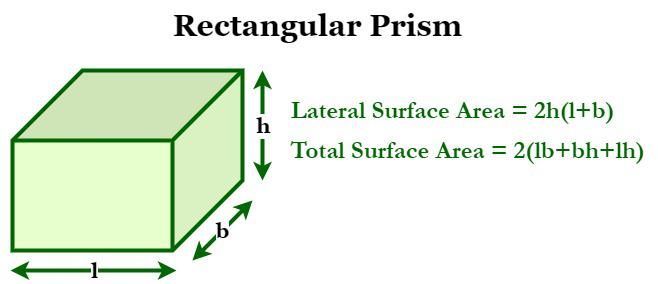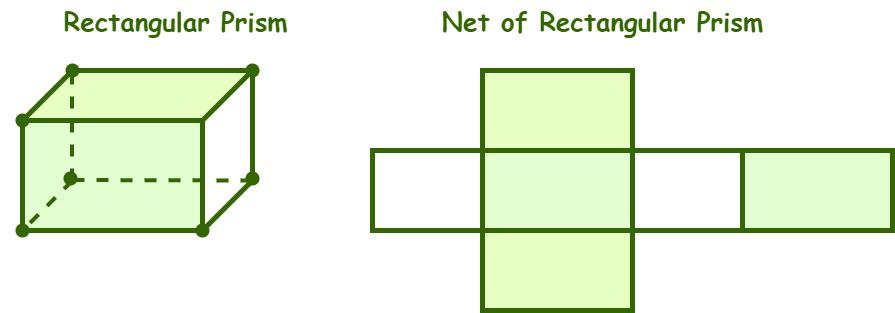GFG App
Open AppBrowser
Continue

# Surface Area of a Rectangular Prism

A rectangular prism is a three-dimensional geometric figure that has four lateral faces with two congruent and parallel bases. A rectangular prism is a polyhedron, and every face is a rectangle. A rectangular prism has a total of six faces where the opposite faces are identical, i.e., a rectangular prism has three pairs of identical faces. The dimensions of a rectangular prism are length, width, and height. It has a total of six faces, twelve edges, and eight vertices. Some examples of rectangular prisms that we see in our everyday lives are fish tanks, notebooks, diaries, cargo containers, rooms, etc.## Surface Area of a Rectangular PrismThe total area occupied by all the three-dimensional surfaces of a three-dimensional geometric structure is called the surface area. The surface area of the prism is equal to the area of its net. So, to find the surface area of a rectangular prism, we have to calculate the areas of each of its faces, then add the resulting areas. A rectangular prism has two types of surface areas: a lateral surface area and a total surface area.

## Surface Area of Rectangular Prism Formula

There are two formulas that are used to calculate the area of a rectangular prism which are,

### Lateral Surface Area Formula

The lateral surface area of a prism (LSA) is equal to the sum of the areas of its four lateral faces.

Lateral Surface Area of a Prism (LSA) = Sum of areas of four lateral faces.

So, the formula for calculating the lateral surface area of a rectangular prism is given as follows:

Lateral Surface Area of a Rectangular Prism = 2h (l + b) square units

where,
l” is the length of the side of a base,
b” is the breadth of the side of a base,
h” is the height of the prism.

### Total Surface Area Formula

The total surface area of a rectangular prism is equal to the sum of the total areas of all its faces.

Total Surface Area of a Prism (TSA) = LSA + 2 × Base area

So, the formula for calculating the total surface area of a rectangular prism is given as follows:

TSA = 2(lb + bh + lh) square units

where,
l” is the length of the side of a base,
b” is the breadth of the side of a base,
h” is the height of the prism.

## How to Find the Surface Area of a Rectangular Prism?

Let us go through an example to understand the concept of calculating the surface area of a rectangular prism.

Example: Calculate the surface area of a rectangular prism if its height is 15 units and the length and breadth of the base are 10 units and 6 units, respectively.

Step 1: Note the dimensions of the given rectangular prism. In the given example, the length and breadth of the rectangular prism’s base are 10 units and 6 units, respectively, and its height is 15 units.

Step 2: We know that the surface area of a rectangular prism is equal to 2(lb + bh + lh) square units. Now, substitute the given values of length, breadth, and height in the formula.

Step 3: So, the surface area of the rectangular prism is calculated as, A = 2× (10 × 6 + 6 × 15 + 10 × 15) = 600 sq. units.

Using the above steps Surface Area of a Rectangular Prism is found.

## Solved Problems on the Surface Area of Rectangular Prism

Problem 1: Determine the total surface area of a rectangular prism if its lateral surface area is 560 sq. cm and the length and breadth of the base are 12 cm and 8 cm, respectively.

Solution:

Given data,

length of the rectangular base (l) = 12 cm

The breadth of the rectangular base (b) = 8 cm

The lateral surface area of the prism (LSA) = 560 sq. cm

We have,

The total surface area of a prism (TSA) = LSA + 2 × Base area

Base area = 2(l + b)

= 2 × (12 + 8) = 2 × 20 = 40 sq. cm

Now, TSA = 560 + 2 × 40

= 560 + 80 = 640 sq. cm

Hence, the rectangular prism’s total surface area is 640 sq. cm.

Problem 2: Calculate the length of the base of a rectangular prism if its height is 9 inches and the breadth of the base is 4 inches, and the lateral surface area is 198 sq. in.

Solution:

Given data,

The lateral surface area = 198 sq. in

The breadth of the rectangular base (b) = 4 inches

Height = 9 inches

length of the rectangular base (l) =?

We have,

The Lateral Surface Area of a Rectangular Prism = 2h (l + b) square units

⇒ 2 × 9 × (l + 4) = 198

⇒ 18 × (l + 4) = 198

⇒ l + 4 = 198/18 = 11

⇒ l = 11 − 4 = 7 in

Thus, the length of the rectangular prism is 7 inches.

Problem 3: Find the lateral surface area of a rectangular prism if its height is 18 cm and the length and breadth of the base are 14 cm and 10 cm, respectively.

Solution:

Given data,

The length of the rectangular base (l) = 14 cm

The breadth of the rectangular base (b) = 10 cm

Height = 18 cm

We know that,

The Lateral Surface Area of a Rectangular Prism = 2h (l + b) square units

= 2 × 18 × (14 + 10)

= 36 × 24 = 864 sq. cm

Hence, the lateral surface of the given prism is 864 sq. cm.

Problem 4: Determine the surface area of a rectangular prism if its height is 12 cm and the length and breadth of the base are 8 cm and 5 cm, respectively.

Solution:

Given data,

The length of the rectangular base (l) = 8 cm

The breadth of the rectangular base (b) = 5 cm

Height = 12 cm

We have,

The Total Surface Area of a Rectangular Prism = 2(lb + bh + lh) square units

= 2 × (8 × 5 + 5 × 12 + 8 × 12)

= 2 × (40 + 60 + 96)

= 2 × 196 = 392 square units

Hence, the rectangular prism’s surface area is 392 square units.

Problem 5: Determine the surface area of a rectangular prism if its height is 14 units and the length and breadth of the base are 10 units and 7 units, respectively.

Solution:

Given data,

The length of the rectangular base (l) = 10 units

The breadth of the rectangular base (b) = 7 units

Height = 14 units

We have,

The Total Surface Area of a Rectangular Prism = 2(lb + bh + lh) square units

= 2 × (10 × 7 + 7 × 14 + 10 × 14)

= 2 × (70 + 98 + 140)

= 2 × 308 = 616 square units

Hence, the rectangular prism’s total surface area is 616 square units.

## FAQs on Rectangular Prism

Question 1: What is meant by a rectangular prism?

In mathematics, a rectangular prism is a three-dimensional geometric figure that has four lateral faces with two congruent and parallel bases. The dimensions of a rectangular prism are length, width, and height. It has a total of six faces, twelve edges, and eight vertices.

Question 2: Mention some examples of a rectangular prism.

Some examples of rectangular prisms that we see in our everyday lives are fish tanks, notebooks, diaries, cargo containers, rooms, etc.

Question 3: What is the total surface area of a rectangular prism?

The total surface area of a rectangular prism is equal to the sum of the total areas of all its faces.

TSA = 2(lb + lh + bh) square units

Where “l” is the length of the side of a base, “b” is the breadth of the side of a base, and “h” is the height of the prism.

Question 4: What is the lateral surface area of a rectangular prism?

The lateral surface area of a prism (LSA) is equal to the sum of the areas of its four lateral faces.

So, the formula for calculating the lateral surface area of a rectangular prism is given as follows:

LSA = 2h (l + b) square units

Where “l” is the length of the side of a base, “b” is the breadth of the side of a base, and “h” is the height of the prism.

### Related Articles

My Personal Notes arrow_drop_up